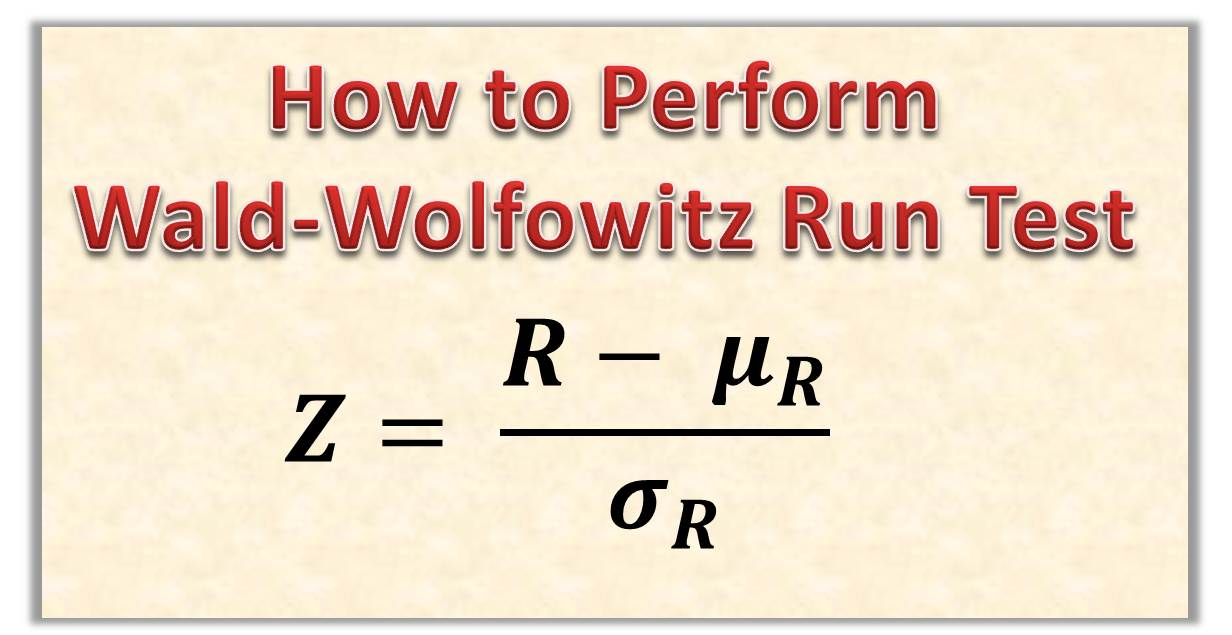# How to Perform Wald-Wolfowitz Test – Testing for Homogeneity with Run TestToday, we are going to go through the steps of performing the Wald-Wolfowitz test (run test). Remember, the easiest way to understand hypothesis testing is to solve an example. So instead of boring you with explanations, we would solve an example together. I would be explaining as we solve.

Content

#### 1. What is Wald-Wolfowitz Test?

Wald-Wolfowitz Test (also called Wald-Wolfowitz run test) is a non-parametric hypothesis test used to test the randomness of a two-valued data sequence. It tests to see if the sequence are mutually independent.

#### 2. Formula for Wald-Wolfowitz Test

The three formulas for the Wald-Wolfowitz run test  are given below:

where the mean is given by the formula

and the variance is given by the formula

Note: Variance is the square of the standard deviation. So we calculated variance. To get the standard deviation, we must take the square root of the variance.

Let’s now solve an example!

#### 3. Example 1

There are two IVM(Innoson Vehicle Manufacturers) buses, one with 48 passengers,  and another with 38 passengers.
Let X and Y denote the number of miles travelled per day for the 48-passenger and 38-passenger buses respectively. Innoson would like to test the equality of the two distributions.
That is, if:

H0: F(z) = G(z)

The company observed the following data on a random sample of n1 = 10 buses carrying 48 passengers and n2 = 11 buses carying 38 passengers.

X: 104 253 300 308 315 323 331 396 414 452
Y:  184 196 197 248 260 279 355 386 393 432 450

Using normal approximation to R, conduct a Wald-Wolfowitz test at 0.05 level of significance

#### 4. Solution

We would solve this problem step by step.

##### Step 1: State the null and the alternate hypothesis and rejection criteria

H0: F(z) = G(z)
H1: F(z) = G(z)

Rejection criteria: Reject the null hypothesis if

##### Step 2: Merge the two lists and sort in ascending order

104  184  196  197  248  253  260  279  300  308  315  331  355  386  393  394  414  432  450  452

##### Step 3: Count the number of runs: R, n1 and n2

Number of runs R = 9
n1 = 10
n2 =  11

##### Step 4: Calculate the mean

We calculate the mean using the formula and we have the results below

##### Step 5: Calculate the variance

We calculation the variance using the calculation steps below

##### Step 6: Calculate Z

We calculate the value of Z following the formula below:

Note: Used 9.5 instead of 9  because we applied half-unit correction for continuity

#### Step 7: Draw your conclusion

We fail to reject the null hypothesis at the 0.05 level because the P value is greater than 0.05. This means that there is not sufficient evidence at 0.05 level to conclude that the two distribution functions are not equal.

Thanks for your effort in learning statistics. If you have any challenge, let me know in the comment box below.